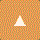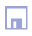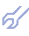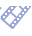Inductive Computer Advisor for Current Forecasting
of Ukrainian Macroeconomy

Initial variables set which characterizes macroeconomy of Ukraine is chosen according to recommendations of economists. Using inductive Combinatorial algorithm the optimal, most accurate non-physical forecast models for four output variables are received. Lead time of forecast is equal to one quarter in this investigation. Not only optimal models are found, but necessary input factors given for normative forecasting are pointed out. High accuracy of short-term forecasting shows possibility of long-term stepwise forecasting in the system under investigation.

Forecasting of processes in macroeconomy has normative character i.e. corresponds to rule "if-then", and because of that may be used for computer-advisor construction, for use in decision making for current regulation of macroeconomy. Normative forecastings are received by GMDH polynomial algorithms, in result of observations of system work for several years proceeding. For example, for investigation of present-day Ukraina macroeconomy the data for 1992-1995 years are used.

Solution of following questions is important for problem answer:

• which full set of variables is available for computer for synthesis and sorting of forecasting models by external criterion. Experience of economists investigating the system should be used, because of great number of variables. But sorting of variants of variables sets is helpful too. That set is better for which forecasting error variance criterion minimum RRmin is smaller;
• which variables are included into input factors subset, and which into subset of output optimized variables. Input factors of normative forecast are chosen usually among manipulated variables of macroeconomic control system. Input factors are used as arguments of forecasting non-physical models. Manipulated control variables are used as arguments of a physical models of object. Models and purposes of forecasting and control are different. Therefore the sets of input factors and manipulated variables can be different too;
• which constrains are given on maximum values of input factors and output variables.

In this subsection is presented, on example of Ukraina, the general method of normative forecasting of processes in macroeconomy, for observation and control aims. There is shown one variant of full sets of input factors and output variables sets only. No doubt it should be interesting too, same other variants, which use other sets of factors and output variables.

### Initial material characteristic and set of variables choice

Let us denote input factors of normative forecast by xi (1<i<M), output variables by yj (1<j<L) and external disturbances by zs (1<s<K)

In we find that macroeconomic systems are characterised by values of following basic variables:

y1 - Real Internal Whole Product (IWP in billions of karbovanets of 1991};
y2 - Consumer prices inflation (CPI);
y3 - Budget deficit (in % of IWP);
y4 - Unemployment (thousands of people);
x1 - Monetary base increase (in % of IWP);
x2 - Number of privatised plants;
x3 - Consumer price index;
x4 - Monetary circulation rate
z1 - Gryvna course for non-trade operations (to US dollar)

Averaged for quarters values of variables for 13 quarters of 1992-1995 are presented in table.

 Year and quarter Y1 Y2 Y3 Y4 X1 X2 X3 X4 Z1 1992 Q1 86.4 3.0 24.1 13.2 13.2 5 75.4 65.1 0.002 Q2 107.6 8.1 35.6 11.1 11.1 10 18.1 100 0.002 Q3 97.5 9.3 60.7 18.5 18.5 20 19.2 123.3 0.003 Q4 96.4 17.7 70.5 45.2 45.2 30 28.5 81 0.008 1993 Q1 84.7 1.1 79.5 32.4 32.4 430 39.7 54.3 0.019 Q2 78.9 13.2 73.3 35.9 35.9 830 39.4 38.2 0.031 Q3 72.3 6.2 78.7 27.7 27.7 1685 44.5 38.9 0.083 Q4 56.4 6.1 83.9 12.2 12.2 3585 66.4 20.6 0.267 1994 Q1 38.8 6.4 98.6 11.8 11.8 5442 12.4 26.8 0.356 Q2 42.4 8.9 92.8 12.9 12.9 8402 5.0 33.3 0.434 Q3 46.9 19.8 88.9 22.6 22.6 10214 4.0 45.7 0.466 Q4 44.5 8.1 82.2 7.3 7.3 11552 39.5 25.4 1.102 1995 Q1 36.2 8.0 86.6 3.7 3.7 12802 16.8 22.8 1.427

It is convenient to transform data to dimension-free form by variables normalization formulae:

Yj = (yj-yjmin)/(yjmax-yjmin); Xi=(xi-ximin)/(ximax-ximin)

Difference forecasting optimal non-physical models for explicit patterns

By pattern is called the graph which shows delayed arguments proposed to computer selection choice. The use of implicit patterns leads to accuracy increase, but connected with a system of linear equations solution for each point of normative forecasting. For explicit patterns forecasting accuracy is smaller, but forecasting models are not linked, calculations are simpler. For first variable Y1(k) forecasting, using explicit pattern (b), Fig.1. we can complete full regression equation in following form:

Y1(k) = a00+a11 Y1(k-1) + a12 Y1(k-2)+ a21 Y2(k-1) + a22 Y2(k-2) + a31 Y3(k) + a32 Y3(k-2)
+ a41 Y4(k-1) + a42 Y4(k-2)+ a50 X1(k) + a51 X1(k-1) + a52 X1(k-2)
+ a60X2(k) + a61 X2(k-1) + a62 X2(k-2) + a70 X3(k) + a71 X3(k-1) + a72 X3(k-2)
+ a80 X4(k) + a81 X4(k-1) + a82 X4(k-2) + a90 Z1(k) + a91 Z1(k-1) + a92 Z1(k-2)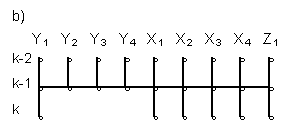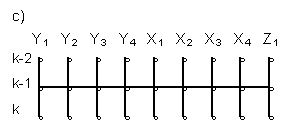Fig. 1. Patterns:

1. explicit, for variable Y1 step-wise forecasting;
2. explicit, for normative Y1(k) forecasting;
3. implicit for normative of Y1(k) Y2(k) Y3(k) and Y4(k) forecasting;

Full equations of analogical form were completed for output variables Y2(k), Y3(k) and Y4(k) too. Then, using Combinatorial GMDH algorithm, coefficients for these equations were found. Forecastings were calculated and their accuracy was evaluated.

An example of normative forecasting

For example, let us consider normative forecasting of Ukraina macroeconomy on second quarter of 1995 using data presented in table. Variables having k index will be related to this quarter. Following values of coefficients were received for the model forecasting Y1(k) :

a00=-0.823; a11= 0; a122= 0; a20= 0; a21=-1.527; a22=-1.539;
a30= 0; a31= 0.549; a32= 0; a40= 0 a41=-0.120 a42= 0.798
a50= 0 a51= 0 a52= 0.295; a60= 1.226; a61=-0.800; a62= 0;
a70= 1.311; a71= 0; a72=-0.581; a80= 0.860; a81= 0; a82= 2.121;
a90= 0.734; a91=-0.467; a92= 2.364

Models accuracy can be characterised by minimal value of RR criterion. For optimal non-physical forecasting model of Y1(k) was RRmin=1.59e-14 (MSE=0.0). For forecast of variable Y2(k) RRmin=7.55e-14 (MSE=3.92e-15); for forecast of Y3(k) RRmin = 3.63e-5 (MSE=6.77e-6); and for forecast of Y4(k) RRmin=0.0026 (MSE=1.05e-4). Small minimal value of criterion shows high accuracy of variable Y1(k) forecasting.

Arguments which have zero coefficient are excluded from model. This means only that these arguments should be not used for accurate forecasting. Conclusions about reasonable use of them for control purposes are not true. For solution of questions about control the analysis of other, physical model (which us not considered here) is necessary.

Values of all variables for delayed moments k-1 and k-2 are known. Substituting them into equations for variables Y1(k), Y2(k), Y3(k) and Y4(k) for explicit patterns we receive four separated calculating equations:

Y1(k) = a0 + a1 X1(K) + a2 X2(k) + a3 X3(k) + a4 X4(k) + a5 Z1(k) (1)
Y2(k) = b0 + b1 X1(k) + b2 X2(k) + b3 X3(k) + b4 X4(k) + b5 Z1(k) (2)
Y3(k) = c0 + c1 X1(k) + c2 X2(k) + c3 X3(k) + c4 X4(k) + c5 Z1(k) (3)
Y4(k) = d0 + d1 X1(k) + d2 X2(k) + d3 X3(k) + d4 X4(k) + d5 Z1(k) (4)

a0=-2.213; a1=0; a2=1.226; a3=1.311; a4=0.860; a5=0.734

computer algorithm teaches us that to receive accurate normative forecast of Y1(k) variable is necessary to point out the values of factors X2(k) X3(k) and X4(k). For equation (2) we receive:

b0=0.337; b1=0.564; b2=0; b3=0; b4=1.542; b5=0

For normative forecasting of Y2(k) variable is necessary to point out factors X1(k) and X4(k). For equation (3) we receive:

c0=-0.639; c1=0.088; c2=1.477; c3=0; c4=0; c5=0

For normative forecasting of Y3(k) variable, factors X1(k) and X2(k) should be pointed out. At last, for equation (4) we find:

do=0.003; d1=0; d2=0; d3=0.756; d4=0; d5=0

For the most accurate normative forecasting of Y4(k) variable should be pointed out only one factor X3(k).

Graphical visualisation of variable Y1(k) normative forecasting

Let us show an example of output variable Y1(k) normative forecasting visualisation using example considered above. Let give to variable X4(k) two values:

X4(k) = 0.25 and X4(k) =0.75

The variable Y1(k) change is shown by isolines on the plane of two input factors X2(k) and X3(k) (Fig.2a and Fig.2b).Fig.2. Graphical presentation of variable Y1(k) forecast.

By comparison of Figures 2a and 2b is possible, particularly, to conclude that increase of monetary circulation rate leads to increase of Y1(k); Most important is that using Figures 2a and 2b is possible to establish quantitative connection between output variable and their input factors. Similar figures can be completed for each output variable.